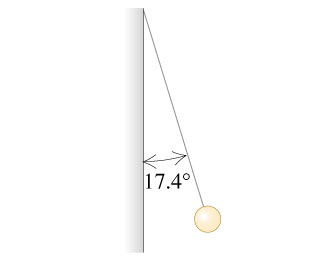# Problem: A small 12.8 g plastic ball is tied to a very light 25.2 cm string that is attached to the vertical wall of a room. (See the figure.) A uniform horizontal electric field exists in this room. When the ball has been given an excess charge of -1.20 μC, you observe that it remains suspended, with the string making an angle of 17.4° with the wall.a) Find the magnitude of the electric field in the room.b) Find the direction of the electric field in the room.

###### FREE Expert Solution

We have three forces acting on the ball: mg, string tension, and electric force.

The electric force must be repelling the ball away from the wall. We'll represent these forces in a free body diagram then decompose any 2D forces into their x and y components. Finally, We'll apply Newton's second law in the x and y-directions.

Newton's second law:

$\overline{){\mathbf{\Sigma }}{\mathbf{F}}{\mathbf{=}}{\mathbf{m}}{\mathbf{a}}}$

Electric force:

$\overline{){\mathbf{F}}{\mathbf{=}}{\mathbf{q}}{\mathbf{E}}}$

2D vector components:

where θ is the angle measured from the x-axis.

97% (176 ratings)###### Problem Details

A small 12.8 g plastic ball is tied to a very light 25.2 cm string that is attached to the vertical wall of a room. (See the figure.) A uniform horizontal electric field exists in this room. When the ball has been given an excess charge of -1.20 μC, you observe that it remains suspended, with the string making an angle of 17.4° with the wall.a) Find the magnitude of the electric field in the room.

b) Find the direction of the electric field in the room.

Frequently Asked Questions

What scientific concept do you need to know in order to solve this problem?

Our tutors have indicated that to solve this problem you will need to apply the Relationships Between Force, Field, Energy, Potential concept. You can view video lessons to learn Relationships Between Force, Field, Energy, Potential. Or if you need more Relationships Between Force, Field, Energy, Potential practice, you can also practice Relationships Between Force, Field, Energy, Potential practice problems.